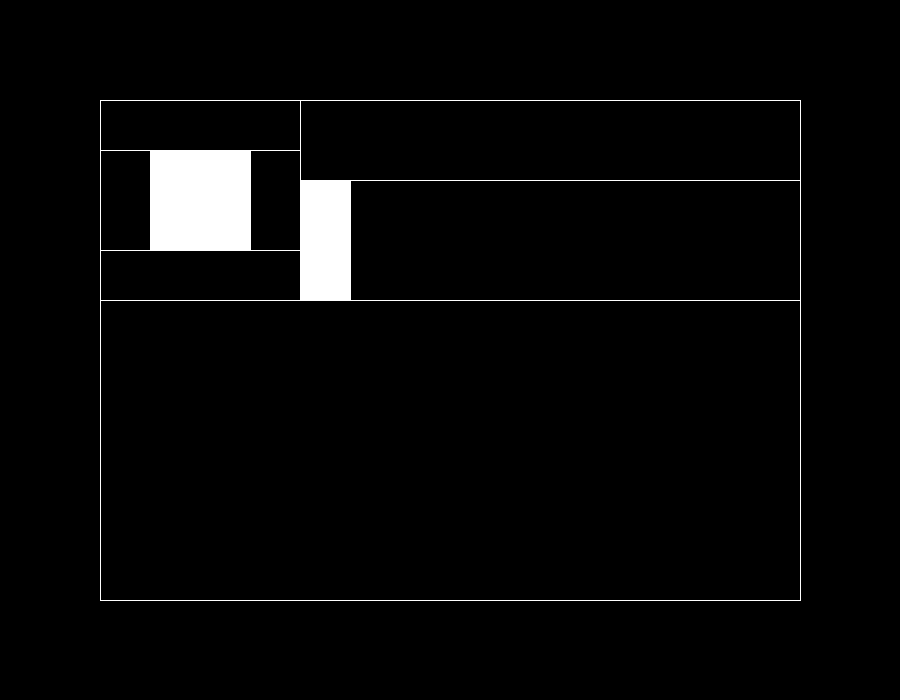Fenrier Lab

# 算法题：矩形分割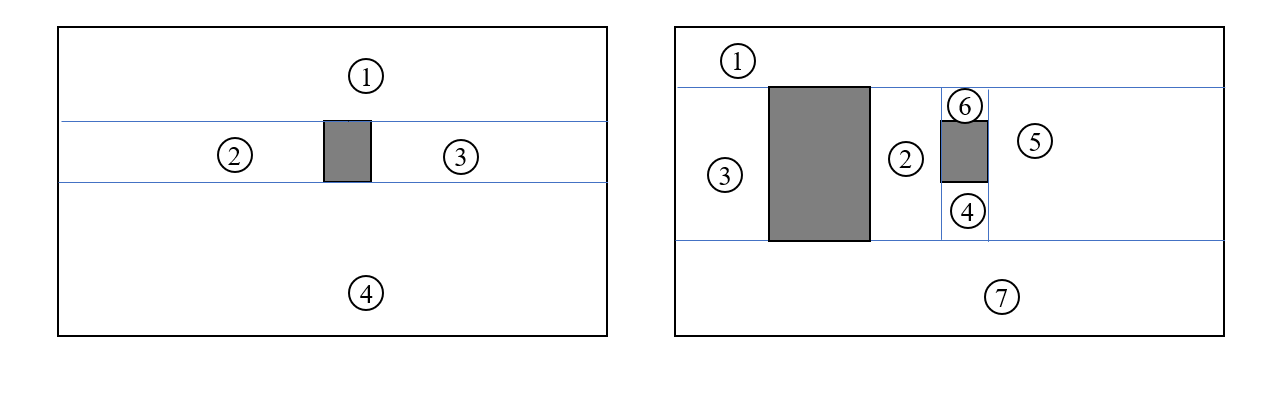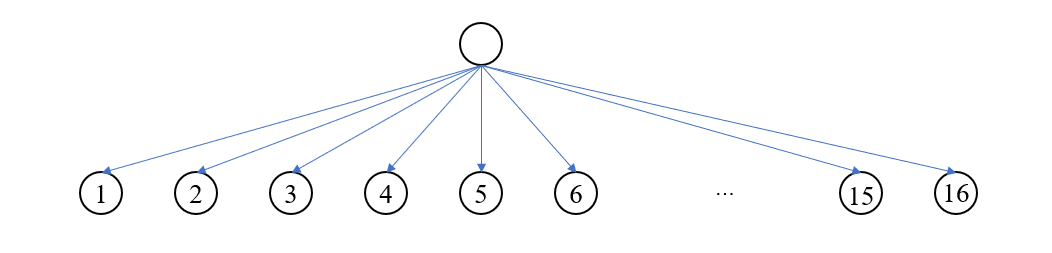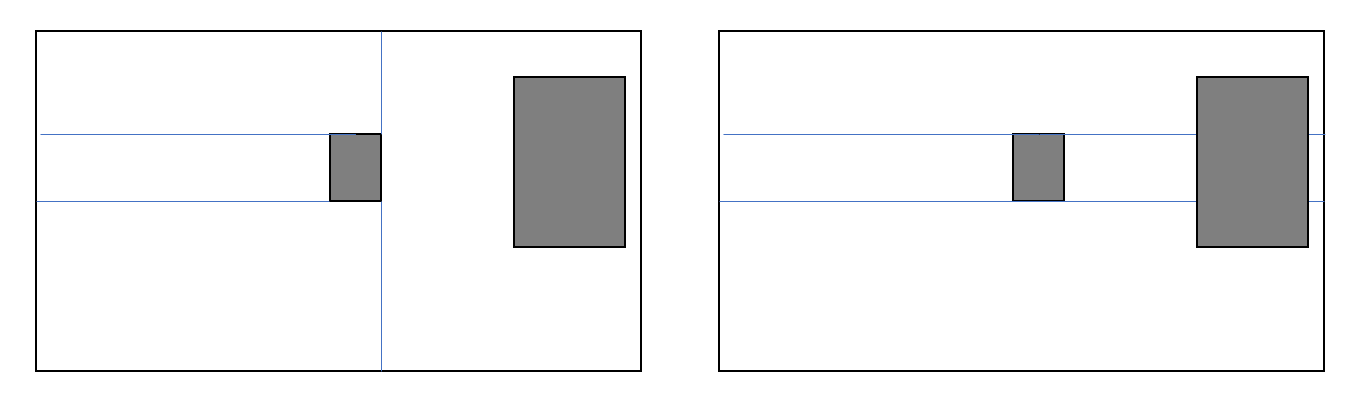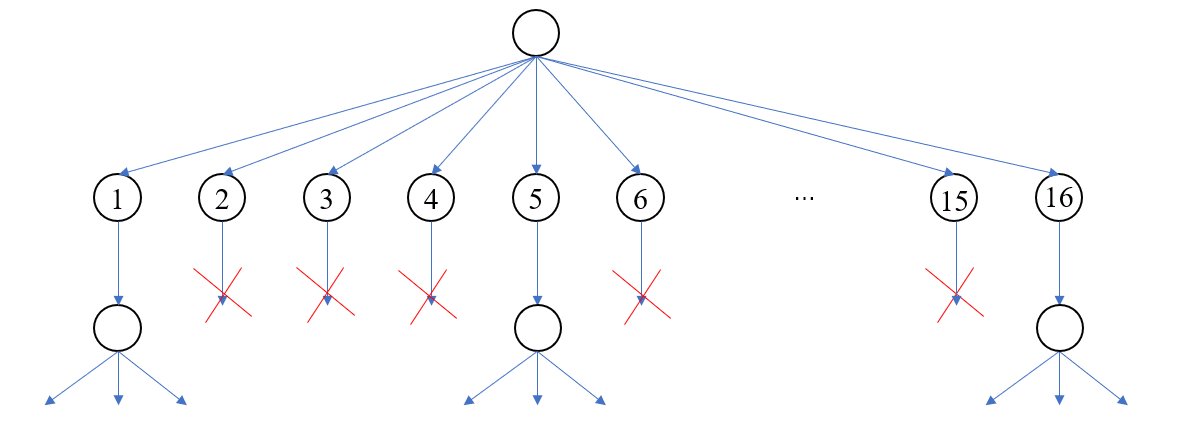/**
* 矩形被方块分割成 4 个矩形
* @param rect 背景矩形坐标
* @param obj 方块坐标
* @param axisDirection 方块四个点的分割方向，0代表横向，1代表纵向
* @return 四个子区域的坐标
* */
public static List<int[]> splitRect(int[] rect, int[] obj, int[] axisDirection) {

int left = 0;
int right = 1;
int top = 2;
int bottom = 3;

int l = rect[left];
int r = rect[right];
int t = rect[top];
int b = rect[bottom];
int ol = obj[left];
int or = obj[right];
int ot = obj[top];
int ob = obj[bottom];
int[][] rects = new int;
rects = new int[]{l, ol, t, b};
rects = new int[]{l, r, t, ot};
rects = new int[]{or, r, t, b};
rects = new int[]{l, r, ob, b};
if(axisDirection == 0) {
rects[top] = obj[top];
rects[bottom] = obj[top];
}else {
rects[right] = obj[left];
rects[left] = obj[left];
}

if(axisDirection == 0) {
rects[bottom] = obj[top];
rects[top] = obj[top];
}else {
rects[right] = obj[right];
rects[left] = obj[right];
}

if(axisDirection == 0) {
rects[bottom] = obj[bottom];
rects[top] = obj[bottom];
}else{
rects[left] = obj[right];
rects[right] = obj[right];
}

if(axisDirection == 0) {
rects[bottom] = obj[bottom];
rects[top] = obj[bottom];
} else {
rects[right] = obj[left];
rects[left] = obj[left];
}

return Arrays.asList(rects);
}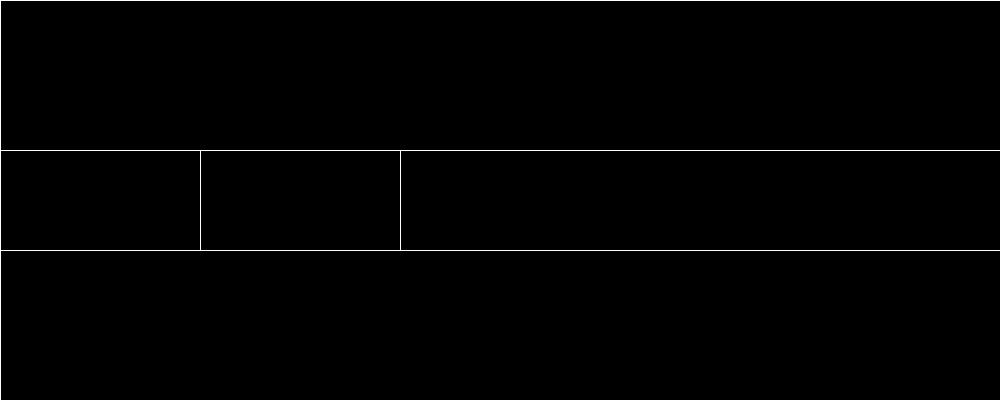/**
* 给定数字 0...n-1，求由这些数字组成长度为 len 的数组的全排列
* 终止解：给定数字 0...n-1，由这些数字组成长度为 1 的数组的全部情况为 , , ... [n-1]
* 递归问题：给定数字 0...n-1，以及由这些数字组成长度为 len - 1 的数组的全排列，求由这些数字组成长度为 len 的数组的全排列
* */
public static List<List<Integer>> permutation(int len, int n) {

// 终止条件
if(len == 1) { // 构造列表 [, , ... [n-1]]
ArrayList<List<Integer>> list = new ArrayList<>();
for (int i = 0; i < n; i++) {
ArrayList<Integer> ls = new ArrayList<>();
}
return list;
}

// 递归求解子问题
List<List<Integer>> list = permutation(len - 1, n);

return list.stream()
.flatMap(ls -> {
ArrayList<List<Integer>> lists = new ArrayList<>();
for (int i = 0; i < n; i++) {
ArrayList<Integer> ls2 = new ArrayList<>(ls);
}
return lists.stream();
})
.collect(Collectors.toList());

}


  /**
* 判断两个矩形是否有交集
* 如果某一个矩形的左边大于另一个矩形的右边，或某矩形的上边小于另一矩形的下边，则两者不相交，否则相交
* */
def isRectOverlap(rect1: Array[Int], rect2: Array[Int]): Boolean = {

!(rect1(0) > rect2(1) || rect1(1) < rect2(0) || rect1(2) > rect2(3) || rect1(3) < rect2(2))

}

/**
* 判断两个矩形是否是包含关系
*
* @param rect1 外部矩形
* @param rect2 内部矩形
* */
def isRectContains(rect1: Array[Int], rect2: Array[Int]): Boolean = {

rect1(0) < rect2(0) && rect1(1) > rect2(1) && rect1(2) < rect2(2) && rect1(3) > rect2(3)

}



/**
* 给定一个矩形，以及 n 个方块，求解对矩形的所有分割方案
* 终止解：给定一个矩形，以及一个方块，可以把矩形分割成4个小矩形区域，且共有16种方案
* 递归问题：已知一个矩形，以及 n - 1 个方块对其进行分割的所有方案，求解再添加一个方块得到的所有切割方案
* @param rect 被分割矩形
* @param objs 方块列表
* */
public static List<List<int[]>> splitRect(int[] rect, List<int[]> objs) {

if(objs.isEmpty()) {
ArrayList<List<int[]>> lists = new ArrayList<>();
ArrayList<int[]> list = new ArrayList<>();
return lists;
}

if(objs.size() == 1) {
return splitRect(rect, objs.get(0));
}

int[] obj = objs.remove(0);

// 求解子问题
List<List<int[]>> plans = splitRect(rect, objs);

// 向目前的分割方案中添加一个方块
return plans.stream()
.flatMap(plan -> {

// 判断当前方块是否与现有区域相交
boolean intersect = plan.stream()
.anyMatch(rt -> isRectOverlap(rt, obj) && !isRectContains(rt, obj));

if(intersect) {
return Stream.empty();
}

// 找到包含当前方块的区域，用于后续分割
Optional<int[]> contains = plan.stream()
.filter(rt -> isRectContains(rt, obj))
.findFirst();
if(!contains.isPresent()) {
return Stream.empty();
}

// 不包含当前方块的区域列表
List<int[]> plan2 = plan.stream()
.filter(rt -> !isRectContains(rt, obj))
.collect(Collectors.toList());
int[] rt = contains.get();

return splitRect(rt, obj)
.stream()
.map(list -> {
List<int[]> newPlan = new ArrayList<>(plan2);
return newPlan;
});
})
.filter(list -> !list.isEmpty())
.collect(Collectors.toList());

}


ArrayList<int[]> objs = new ArrayList<>();
int[] r1 = {150, 250, 150, 250};